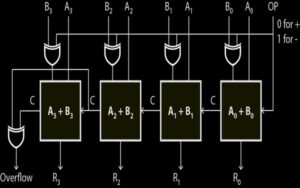# VHDL Code for 4-bit Adder / Subtractor

This example describes a two input 4-bit adder/subtractor design in VHDL. The design unit multiplexes add and subtract operations with an OP input. 0 input produce adder output and 1 input produce subtractor output.### VHDL Code for 4-bit Adder / Subtractor

```--FULL ADDER
library ieee;
use ieee.std_logic_1164.all;
port( X, Y, Cin : in std_logic;
sum, Cout : out std_logic);

begin
sum <= (X xor Y) xor Cin;
Cout <= (X and (Y or Cin)) or (Cin and Y);
end bhv;
========================================================
library ieee;
use ieee.std_logic_1164.all;
port( OP: in std_logic;
A,B  : in std_logic_vector(3 downto 0);
R  : out std_logic_vector(3 downto 0);
Cout, OVERFLOW : out std_logic);

port( X, Y, Cin : in std_logic;
sum, Cout : out std_logic);
end component;
signal C1, C2, C3, C4: std_logic;
signal TMP: std_logic_vector(3 downto 0);

begin
TMP <= A xor B;
FA0:Full_Adder port map(A(0),TMP(0),OP, R(0),C1); – R0
FA1:Full_Adder port map(A(1),TMP(1),C1, R(1),C2); – R1
FA2:Full_Adder port map(A(2),TMP(2),C2, R(2),C3); – R2
FA3:Full_Adder port map(A(3),TMP(3),C3, R(3),C4); – R3
OVERFLOW <= C3 XOR C4 ;
Cout <= C4;
end struct;```

### 2 thoughts on “VHDL Code for 4-bit Adder / Subtractor”

1.Hi thanks for the example.
Quick question: why is line 39 included as I was under the impression that C4/Cout was already classed as the overflow, so why include it at all and include C3 as an overflow ??

Thanks

2.